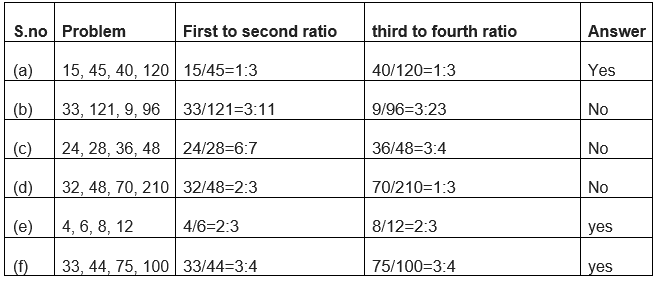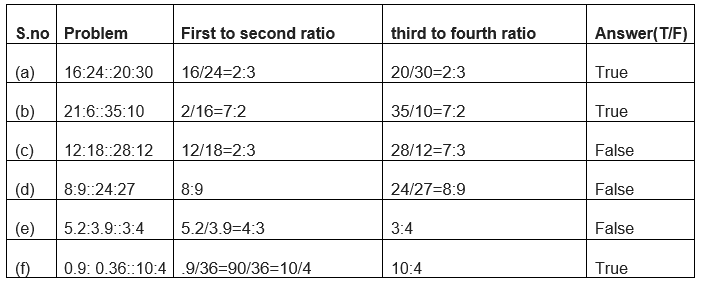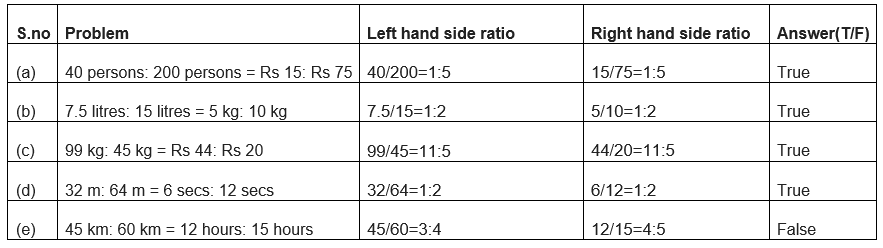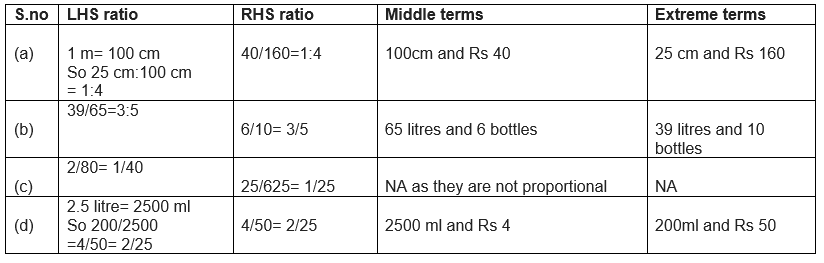# Ratio and proportion Exercise 12.2 and 12.3

In this page we have NCERT Solutions for Class 6 Maths Ratio and proportion Chapter 12 Exercise 12.2 and 12.3. Hope you like them and do not forget to like , social share and comment at the end of the page.

## Exercise 12.2

Question 1
Determine if the following are in proportion.
(a) 15, 45, 40, 120
(b) 33, 121, 9, 96
(c) 24, 28, 36, 48
(d) 32, 48, 70, 210
(e) 4, 6, 8, 12
(f) 33, 44, 75, 100
If the ratio of first to the second is equal to ratio to third to the fourth, these will be in proportionQuestion 2
Write True (T) or False (F) against each of the following statements:
(a) 16:24::20:30
(b) 21:6::35:10
(c) 12:18::28:12
(d) 8:9::24:27
(e) 5.2:3.9::3:4
(f) 0.9:0.36::10:4

If the ratio of first to the second is equal to ratio to third to the fourth, these will be in proportionQuestion 3
Are the following statements true?
(a) 40 persons: 200 persons = Rs 15: Rs 75
(b) 7.5 litres: 15 litres = 5 kg: 10 kg
(c) 99 kg: 45 kg = Rs 44: Rs 20
(d) 32 m: 64 m = 6 secs: 12 sec
(e) 45 km: 60 km = 12 hours: 15 hoursQuestion 4
Determine if the following ratios form a proportion. Also, write the middle terms and extreme terms where the ratios form a proportion.
(a) 25 cm: 1 m and Rs 40: Rs 160
(b) 39 litres: 65 litres and 6 bottles: 10 bottles
(c) 2 kg: 80 kg and 25 g: 625 g
(d) 200 mL: 2.5 litre and Rs 4: Rs 50

When the units are different in one ratio, we need to convert the bigger unit into smaller unit## Exercise 12.3

Question 1
If the cost of 7 m of cloth is Rs 294, find the cost of 5 m of cloth.
In these type of question, we need to find the value per unit value which is find by dividing the quantity given
Cost of 7 m of cloth = Rs 294
So, the cost of 1 m of cloth = ${294}{7}$ = Rs 42
Cost of 5 m of cloth = $42 \times 5$= Rs 210

Question 2
Ekta earns Rs 1500 in 10 days. How much will she earn in 30 days?
Amount Ekta earns in 10 days = Rs 1500
So, amount earned in 1 day by Ekta = Rs 1500 ÷ 10 = Rs.
Money she will earn in 30 days = Rs. 150 × 30 = Rs. 4500

Question 3
If it has rained 276 mm in the last 3 days, how many cm of rain will fall in one full week (7 days)? Assume that the rain continues to fall at the same rate.
Amount of rain in 3 days = 276 mm = 27.6 cm
So, Amount of rain in 1 day = 27.6 ÷ 3 = 9.2 cm
We know that one week has 7 days.
So, Amount of rain in 7 days =$9.2 \times 7 = 64.4 cm$

Question 4
Cost of 5 kg of wheat is Rs 30.50.
(a) What will be the cost of 8 kg of wheat?
(b) What quantity of wheat can be purchased in Rs 61?
Given
Cost of 5 kg of wheat = Rs. 30.50
So, Cost of 1 kg = Rs. 30.50 ÷ 5 = Rs. 6.10
a) Cost of 8 kg of wheat = 8 × (cost of 1 kg of wheat)=8 × Rs. 6.10 = Rs. 48.80
b) Amount of wheat purchased for Rs. 6.10 = 1 kg
Amount of wheat purchased for Rs 61 = 61 ÷ 6.10 = $\frac {6100}{610} = 10 \; kg$

Question 5
The temperature dropped 15 degrees Celsius in the last 30 days. If the rate of temperature drop remains the same, how many degrees will the temperature drop in the next ten days?
Temperature drop in 30 days = 15°C
Rate of Temperature drop in 1 day = 15 ÷ 30 = (½)°C
Temperature drop in 10 days = (½)°C × 10 = 5°C

Question 6
Shaina pays Rs 7500 as rent for 3 months. How much does she has to pay for a whole year, if the rent per month remains same?
Rent paid by Shaina for 3 months = Rs 7500
Rent paid by Shaina per month = 7500 ÷ 3 = Rs 2500
We know that in a year, there are 12 months.
So, the rent paid by Shaina for whole year = $2500 \times 12$ = Rs 30,000

Question 7
Cost of 4 dozen bananas is Rs 60. How many bananas can be purchased for Rs 12.50?
We know that
1 dozen = 12
So, 4 dozen contain 48 bananas
Now The cost of 48 bananas is given as   Rs. 60
So Cost of 1 banana= 60 ÷ 48 = 5 ÷ 4 = Rs 1.25
Number of bananas purchased in Rs 12.50 = $\frac {Total \; Rupees}{ Price \; of \; one \; banana}$
$= \frac {12.5}{ 1.25} = 10$

Question 8
The weight of 72 books is 9 kg. What is the weight of 40 such books?
Given
Weight of 72 books = 9 kg
So, Weight of 1 book = 72 ÷ 9 = 8 Kg
Now Weight of 40 books = $40 \times 8 = 320 \; kg$

Question 9
A truck requires 108 litres of diesel for covering a distance of 594 km. How much diesel will be required by the truck to cover a distance of 1650 km?
Amount of Diesel required by truck for covering a distance for 594 km = 108 l
Amount of Diesel required by truck for covering a distance for   1 km = 108 ÷ 594 = (2/11) litre
So, Amount of diesel required by truck for covering a distance   for 1650 km = 1650 × (2/11) =300 Litre

Question 10
Raju purchases 10 pens for Rs 150 and Manish buys 7 pens for Rs 84. Can you say who got the pens cheaper?
Given
Amount paid by Raju for 10 pens = Rs. 150
Amount paid by Manish for 7 pens = Rs. 84
Therefore
Amount paid by Raju for 1 pen = 150 ÷ 10 = Rs 15
Amount paid by Manish for 1 pen = 84 ÷ 7 = Rs 12
Raju paid Rs 15 for 1 pen but Manish paid Rs. 12
So, Manish got the pens cheaper.

Question 11
Anish made 42 runs in 6 overs and Anoop made 63 runs in 7 overs. Who made more runs per over?
Given
Runs made by Anish in 6 overs = 42
Runs made by Anoop in 7 overs = 63
Therefore
Runs made by Anish in 1 over = 42 ÷ 6 = 7
Runs made by Anoop in 1 over = 63 ÷ 7 = 9
In one over, Anoop made 9 runs but Anish made 7 runs.
So, Anoop made more runs in an over than Anish.### Practice Question

Question 1 What is $\frac {1}{2} + \frac {3}{4}$ ?
A)$\frac {5}{4}$
B)$\frac {1}{4}$
C)$1$
D)$\frac {4}{5}$
Question 2 Pinhole camera produces an ?
A)An erect and small image
B)an Inverted and small image
C)An inverted and enlarged image
D)None of the above